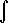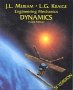# Physics - Conservation laws and Symmetry

### Energy

Energy is a quantity that is constant, that is it does not change with time, for any closed system.

Energy is a scalar quantity, that is it is independent of direction.

Energy can exist in different forms and can sometimes be exchanged between these forms, examples of types of energy are:

#### Potential energy

Energy by virtue of being on a force field. for example gravity, electric and magnetic fields have the potential to do work.

#### Kinetic energy

Energy by virtue of motion, for a particle it is 0.5 * m * v2

where:

• m = mass (scalar quantity)
• v = velocity (we can use the scalar modulus of velocity or if we use multivectors squaring a velocity vector will give a scalar quantity)

For a solid body it is more complicated because rotating solid objects also have kinetic energy, the kinetic energy of a rotating object can be calculated by summing the linear energy of all its particles. However we cannot get the total kinetic energy of a solid body just add the kinetic energy due to rotation to the kinetic energy due to linear motion. We must add another factor to give this equation:

T = 0.5 m v2 + 0.5 wt [I] w + m(v•(w x r))

this is derived here.

#### Heat energy

At the molecular level this is the kinetic energy of the particles that make up the object, so this is a form of kinetic energy.

#### Wave energy

Such as sound, light, water waves. This is due to oscillation between other types of energy.

#### Chemical energy

This is a form of potential energy due to the molecular bonds.

#### Electrical energy

The kinetic energy of electrons in an electric field.

#### Mass

Since we are talking about classical mechanics on most of this site then we usually assume that mass is constant. However, if we need to take into account the effects of Einstein's special relativity then energy can be converted into mass and mass into energy given by E=m c2

### Work

One definition of energy is the ability to do work, where work is force times distance:

work =force • d displacement

where:

• • = dot product
• d = differential operator
• displacement = change in position vector
• force = force vector

I'm not sure this is a strictly valid definition, the second law of thermodynamics suggests that not all forms of energy can be turned into mechanical work?

### Momentum

Momentum is a quantity that is constant for any closed system (like energy).

Momentum is a vector quantity, that is, it has components in x,y and z dimensions (unlike energy) momentum in one dimension cannot be converted to momentum in another dimension, so momentum is conserved in x dimension and it is separately conserved in y and z dimensions.

Momentum cannot be converted to different forms (unlike energy) although momentum can be exchanged between different masses when they interact by means of forces or collisions. For any closed system the total momentum is always constant.

### Angular Momentum

Angular momentum is constant for any closed system (provided that the angular momentum of every object in the system is measured about the same fixed point).

Angular momentum is a vector quantity, it has components about the x,y and z axes (any mutually perpendicular axes) momentum in one dimension cannot be converted to momentum in another dimension, so momentum is conserved in x dimension and it is separately conserved in y and z dimensions.

Angular Momentum is conserved independently of linear momentum. If a force exists between two objects or the objects collide then both angular momentum and linear momentum may be transferred between the objects but neither the angular momentum or the linear momentum of the total system will change. See these examples.

 metadata block see also: Correspondence about this page Book Shop - Further reading. Where I can, I have put links to Amazon for books that are relevant to the subject, click on the appropriate country flag to get more details of the book or to buy it from them.Classical Dynamics of Particles and Systems - I have not reviewed this book, so I would be interested to hear if it is useful? I would also be interested to hear if there are any other books that may be of interest to readers of this page.Engineering Mechanics Vol 2: Dynamics - Gives theory for rigid dynamics, aims to allow prediction of effects of force and motion. Includes rotating frame of reference. Lots of colour diagrams, I guess its college / University level. Commercial Software Shop Where I can, I have put links to Amazon for commercial software, not directly related to the software project, but related to the subject being discussed, click on the appropriate country flag to get more details of the software or to buy it from them.

This site may have errors. Don't use for critical systems.